# US20110081935A1 - Lte-a system and uplink power control method thereof - Google Patents

## Info

Publication number
US20110081935A1
US20110081935A1 US12/896,481 US89648110A US2011081935A1 US 20110081935 A1 US20110081935 A1 US 20110081935A1 US 89648110 A US89648110 A US 89648110A US 2011081935 A1 US2011081935 A1 US 2011081935A1
Authority
US
United States
Prior art keywords
cw
codeword
ue
specific
power control
Prior art date
Legal status (The legal status is an assumption and is not a legal conclusion. Google has not performed a legal analysis and makes no representation as to the accuracy of the status listed.)
Granted
Application number
US12/896,481
Other versions
US8731596B2 (en
Inventor
Myung Hoon YEON
Joon Yong Cho
Jin Kyu Han
Youn Sun KIM
Jianzhong Zhang
Current Assignee (The listed assignees may be inaccurate. Google has not performed a legal analysis and makes no representation or warranty as to the accuracy of the list.)
Samsung Electronics Co Ltd
Original Assignee
Samsung Electronics Co Ltd
Priority date (The priority date is an assumption and is not a legal conclusion. Google has not performed a legal analysis and makes no representation as to the accuracy of the date listed.)
Filing date
Publication date
Priority to KR10-2009-0094044 priority Critical
Priority to KR20090094044 priority
Priority to KR20090105405 priority
Priority to KR10-2009-0105405 priority
Priority to KR10-2009-0107207 priority
Priority to KR20090107207 priority
Priority to KR1020100039186A priority patent/KR20110036484A/en
Priority to KR10-2010-0039186 priority
Priority to KR1020100057939A priority patent/KR20110036489A/en
Priority to KR10-2010-0057939 priority
Priority to KR10-2010-0063747 priority
Priority to KR1020100063747A priority patent/KR101632626B1/en
Application filed by Samsung Electronics Co Ltd filed Critical Samsung Electronics Co Ltd
Assigned to SAMSUNG ELECTRONICS CO., LTD. reassignment SAMSUNG ELECTRONICS CO., LTD. ASSIGNMENT OF ASSIGNORS INTEREST (SEE DOCUMENT FOR DETAILS). Assignors: CHO, JOON YOUNG, HAN, JIN KYU, KIM, YOUN SUN, YEON, MYUNG HOON, ZHANG, JIANZHONG
Publication of US20110081935A1 publication Critical patent/US20110081935A1/en
Application granted granted Critical
Publication of US8731596B2 publication Critical patent/US8731596B2/en
Application status is Active legal-status Critical

• 239000010410 layers Substances 0 abstract claims description 140
• 230000001276 controlling effects Effects 0 abstract claims description 9
• 238000004891 communication Methods 0 claims description 6
• 230000000051 modifying Effects 0 claims description 3
• 238000000034 methods Methods 0 description 23
• 238000004364 calculation methods Methods 0 description 18
• 230000037242 Cmax Effects 0 description 12
• 230000011664 signaling Effects 0 description 11
• 230000003252 repetitive Effects 0 description 10
• 230000001965 increased Effects 0 description 9
• 238000005516 engineering processes Methods 0 description 6
• 230000001702 transmitter Effects 0 description 6
• 239000011159 matrix materials Substances 0 description 2
• 230000002829 reduced Effects 0 description 2
• 230000000875 corresponding Effects 0 description 1
• 230000004048 modification Effects 0 description 1
• 238000006011 modification Methods 0 description 1
• 230000000737 periodic Effects 0 description 1
• 230000001603 reducing Effects 0 description 1
• 239000002356 single layers Substances 0 description 1
• 230000003595 spectral Effects 0 description 1
• 230000001960 triggered Effects 0 description 1

## Images

•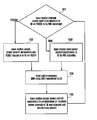•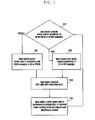•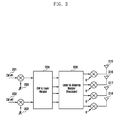•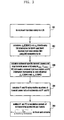•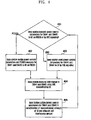•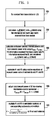•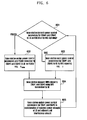•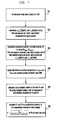•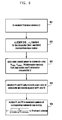•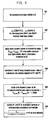•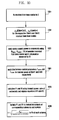•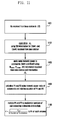•••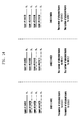•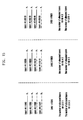## Classifications

• HELECTRICITY
• H04ELECTRIC COMMUNICATION TECHNIQUE
• H04WWIRELESS COMMUNICATION NETWORKS
• H04W52/00Power management, e.g. TPC [Transmission Power Control], power saving or power classes
• H04W52/04TPC
• H04W52/06TPC algorithms
• HELECTRICITY
• H04ELECTRIC COMMUNICATION TECHNIQUE
• H04WWIRELESS COMMUNICATION NETWORKS
• H04W52/00Power management, e.g. TPC [Transmission Power Control], power saving or power classes
• H04W52/04TPC
• H04W52/30TPC using constraints in the total amount of available transmission power
• H04W52/34TPC management, i.e. sharing limited amount of power among users or channels or data types, e.g. cell loading
• H04W52/346TPC management, i.e. sharing limited amount of power among users or channels or data types, e.g. cell loading distributing total power among users or channels

## Abstract

An LTE-A system and power control method for controlling cell interference based on codeword-specific transmission power adjustment with a transmission power control offset parameter. The method includes transmitting a parameter KS signaled by a higher layer and codeword-specific power control parameters from a base station to a terminal; calculating gains at the terminal, using the codeword-specific power control parameters and the parameter KS; calculating, at the terminal, codeword-specific transmit powers according to the gains; and transmitting, from the terminal to the base station, at least two codewords at the codeword-specific transmit powers.

## Description

PRIORITY
• This application claims priority under 35 U.S.C. §119(a) to Korean Patent Application Nos. 10-2009-0094044, 10-2009-0105405, 10-2009-0107207, 10-2010-0039186, 10-2010-0057939, and 10-2010-0063747, which were filed in the Korean Intellectual Property Office on Oct. 1, 2009, Nov. 3, 2009, Nov. 6, 2009, Apr. 27, 2010, Jun. 18, 2010, and Jul. 2, 2010, respectively, the disclosure of each of which is incorporated herein in its entirety by reference.
• BACKGROUND OF THE INVENTION
• 1. Field of the Invention
• The present invention relates generally to wireless communications and, in particular, to a Long Term Evolution-Advanced (LTE-A) system and power control method of the LTE-A system for controlling cell interference by adjusting transmission power per codeword with a transmission power control offset parameter.
• 2. Description of the Related Art
• Long Term Evolution (LTE), i.e., a next generation wireless communication technology, utilizes Orthogonal Frequency Division Multiplexing (OFDM) for downlink transmission and Single Carrier-Frequency Division Multiple Access (SC-FDMA) for uplink transmission.
• In OFDMA, however, Peak to Average Power Ratio (PAPR) is high, which increases a back-off value for an input signal of power amplifier to prevent non-linear distortion of the signal. Accordingly, maximum transmission power is limited as such, resulting in reduced transmission power efficiency. The back-off value limits the maximum value of the transmission power to be less than a value of the power amplifier for guaranteeing the linearity of a transmission signal. For example, if the maximum value of a power amplifier is 23 dBm and a back-off value is 3 dBm, the maximum transmission power is limited to 20 dBm.
• When OFDMA is used as downlink multiplexing technology, there are no problems because the transmitter belongs to a base station having no power limit. However, if OFDMA is used as uplink multiplexing technology, because the transmitter belongs to a User Equipment (UE) that has a very limited in transmission power, the coverage of the base station reduces due to the limit of maximum transmission power of the UE. Therefore, in order to increase uplink coverage, SC-FDMA has been adopted for uplink multiplexing technology of LTE as the 4th generation wireless communication standard of 3rd Generation Partnership Project (3GPP).
• With of the increase use of multimedia services in the wireless communication environment, many researchers are focusing on techniques for achieving high speed transmission. For example, Multiple Input Multiple Output (MIMO) is one of the key techniques for increasing spectral efficiency and link reliability for high speed transmission.
• MIMO technology uses multiple antennas to increase channel capacity in a limited frequency resource. Logically, the channel capacity of MIMO increases in proportion to the number of antennas being used. In order to transmit data efficiently in a MIMO system, the data is encoded in advance, which is commonly referred to as “precoding”. The data precoding rule can be expressed by a precoding matrix, and a set of precoding matrices is commonly referred to as a “codebook”.
• In LTE-A, a MIMO technique using a precoding matrix is recommended as an uplink transmission technology for improving system performance in multiuser environment as well as single-user environment.
• In LTE uplink, event-triggered power control is used for Physical Uplink Shared Channel (PUSCH). In PUSCH, Transmit periodic Power Control (TPC) feedback is not required. PUSCH transmission power at subframe i PPUSCH(i) can be expressed as shown in Equation (1).

• P PUSCH(i)=min{P CMAX,10 log10(M PUSCH(i))+P O PUSCH(j)+α(jPL+Δ TF(i)+f(i)}[dBm]  (1)
• In Equation (1), PCMAX denotes a maximum transmission power according to the UE power class, and MPUSCH(i) denotes a number of Resource Blocks (RBs) as the PUSCH resource assigned in the subframe i. The transmission power of the UE increases in proportion to MPUSCH(i). PL denotes downlink path-loss measured at the UE, and α(j) denotes a scaling factor, which is determined at higher layers in consideration of a difference between uplink and downlink channels established by a cell formation. PO PUSCH(j) can be expressed as shown in Equation (2).

• P O PUSCH(j)=P O NOMINAL PUSCH(j)+P O UE PUSCH(j)  (2)
• In Equation (2), PO NOMINAL PUSCH(j) denotes a cell-specific parameter signaled by a higher layer, and PO UE PUSCH(i) denotes a UE-specific parameter transmitted through Radio Resource Control (RRC) signaling. A Modulation and Coding Scheme (MCS) or Transport Format (TF) compensation parameter ΔTF(i) can be defined as shown in Equation (3).
• $Δ TF  ( i ) = { 10  log 10  ( 2 MPR  ( i ) · K S - 1 ) for   K S = 1.25 0 for   K S = 0 ( 3 )$
• In Equation (3), KS is a cell-specific parameter transmitted through RRC signaling.
• MPR(i) is calculated by Equation (4).
• $M   P   R  ( i ) = T   B   S  ( i ) M PUSCH  ( i ) · N SC RB · 2  N Symb UL ( 4 )$
• In Equation (4), TBS(i) is a transport block size of subframe i, and MPUSCH(i)·NSC RB·2NSymb UL denotes a number of Resource Elements (REs) within the subframe. MPR(i), which is calculated by Equation (4) indicates a number of information bits per RE.
• If KS=0 and MPR(i)=0, then the uplink channels are not compensated for MCS.
• If KS=1.25, only 80%
• $( 1 K S = 0.8 )$
• of the uplink channels are compensated for MCS.
• The current PUSCH power control adjustment state is given by f(i), which is defined by equation (5).

• f(i)=f(i−1)+δPUSCH(i−K PUSCH),  (5)
• In Equation (5), δPUSCH is a UE-specific parameter included in a Physical Downlink Control Channel (PDCCH) transmitted from the base station to the UE and can be a TPC value, and KPUSCH in δPUSCH(i−KPUSCH) denotes a time interval between receiving δPUSCH value and using it in the transmission subframe.
• The δPUSCH dB accumulated values signaled on PDCCH with DCI format 0 are [−1, 0, 1, 3]. The δPUSCH dB accumulated values signaled on PDCCH with DCI format 3/3A are [−1, 1] or [−1, 0, 1, 3].
• Rather than δPUSCH accumulated values as shown in Equation (5), δPUSCH dB absolute values can be used as shown in Equation (6).

• f(i)=δPUSCH(i−K PUSCH)  (6)
• In Equation (6), the δPUSCH dB absolute values signaled on PDCCH with DCI format 0 are [−4, −1, 1, 4].
• FIG. 1 is a flowchart illustrating a base station-assisted UE power control process in a conventional LTE system.
• Referring to FIG. 1, the base station determines whether to use PDCCH or RRC signaling to transmit power control parameters to the UE in step 101.
• If PDCCH is selected (e.g., δPUSCH), the base station sends the power control parameters to the UE on PDCCH in step 102. However, if RRC signaling is selected (KS), the base station sends the power control parameters using RRC signaling in step 103.
• In step 104, the base station measures Signal-to-Interference plus Noise Ratio (SINR) using Sounding Reference Signal (SRS) transmitted by the UE.
• In step 105, the base station updates the power control parameters in consideration of the received signal strength and the interference of the signal, which is transmitted by the UE, to neighbor cells. The updated parameters are transmitted to the UE on the channel determined in step 101.
• In the LTE-A system, two codewords are transmitted on the PUSCH with MIMO. Accordingly, applying the convention power control method designed for the LTE uplink using a single antenna and single codeword to the LTE-A system increases cell interference due to the excessive transmission power of the UE and early transmission power shortage.
• SUMMARY OF THE INVENTION
• Accordingly, the present invention is designed to solve at least the above-described problems of the prior art, and to provide at least the advantages described below.
• An aspect of the present invention is to provide an uplink power control method for a UE with multiple transmit antennas assigned individual codewords in an LTE-A system that is capable of reducing cell interference by controlling transmission powers per codeword.
• Another aspect of the present invention is to provide an uplink power control method for a UE with multiple transmit antennas identified by individual codewords in an LTE-A system, which prevents a cell interference increases when using multiple transmit antennas, by using transmit power control offset parameters that increase transmit power for respective codewords.
• In accordance with an aspect of the present invention, a method for controlling transmit power of a user terminal communicating with a base station using at least two codewords in a wireless communication is provided. The method includes transmitting a parameter KS signaled by a higher layer and codeword-specific power control parameters from the base station to the terminal; calculating, at the terminal, gains using the power control parameters and the parameter KS; calculating, at the terminal, codeword-specific transmit powers according to the gains; and transmitting at least two codewords at the codeword-specific transmit powers from the terminal to the base station.
• In accordance with another aspect of the present invention, a transmit power control system is provided which includes a base station that transmits a cell-specific parameter KS given by a higher layer and codeword-specific power control parameters; and a terminal that receives the cell-specific parameter KS, calculates gains using the power control parameters and the parameter KS, and transmits at least two codewords at codeword-specific transmit powers calculated with the gains to the base station.
• BRIEF DESCRIPTION OF THE DRAWINGS
• The above and other aspects, features, and advantages of the present invention will be more apparent from the following detailed description in conjunction with the accompanying drawings, in which:
• FIG. 1 is a flowchart illustrating a base station-assisted UE power control process in a conventional LTE system;
• FIG. 2 illustrates a transmitter of a UE according to an embodiment of the present invention;
• FIG. 3 is a flowchart illustrating a transmit power calculation process according to an embodiment of the present invention;
• FIG. 4 is a flowchart illustrating a UE-transmit power control method of a base station in an LTE-A system according to an embodiment of the present invention;
• FIG. 5 is a flowchart illustrating a transmit power calculation process according to an second embodiment of the present invention;
• FIG. 6 is a flowchart illustrating a UE-transmit power control method of a base station in an LTE-A system according to an embodiment of the present invention;
• FIG. 7 is a flowchart illustrating a transmit power calculation process according to an embodiment of the present invention;
• FIG. 8 is a flowchart illustrating a transmit power calculation process according to an embodiment of the present invention;
• FIG. 9 is a flowchart illustrating a transmit power calculation process according to an embodiment of the present invention;
• FIG. 10 is a flowchart illustrating a transmit power calculation process according to an embodiment of the present invention;
• FIG. 11 is a flowchart illustrating a transmit power calculation process according to an embodiment of the present invention;
• FIG. 12 is a flowchart illustrating a transmit power calculation process according to an embodiment of the present invention;
• FIG. 13 is a flowchart illustrating a transmit power calculation process according to an embodiment of the present invention;
• FIG. 14 is a diagram illustrating power assignments for layers of a UE in an MU-MIMO system according to an embodiment of the present invention; and
• FIG. 15 is a diagram illustrating power assignments for layers of a UE in an MU-MIMO system according to another embodiment of the present invention.
• DETAILED DESCRIPTION OF EMBODIMENTS OF THE INVENTION
• Various embodiments of the present invention are described in detail below with reference to the accompanying drawings. The same reference numbers are used throughout the drawings to refer to the same or like parts. Additionally, detailed descriptions of well-known functions and structures incorporated herein may be omitted to avoid obscuring the subject matter of the present invention.
• The following terms are defined in consideration of the functionality of the embodiments of the present invention, and may vary according to intentions of a user or an operator or according to usual practice. Therefore, the definitions of the terms must be interpreted based on the entire content of the present specification.
• In the embodiments of the invention described herein, descriptions are directed to OFDM-based wireless communication systems, especially, 3GPP EUTRA (or LTE) or Advanced E-UTRA (or LTE-A) systems, however, it is obvious to those skilled in the art that various changes may be made without departing from the scope of the present invention.
• In accordance with an embodiment of the present invention, an uplink power control method for an LTE-A system is provided, in which a UE performs transmission with two transmit antennas assigned different CodeWords (CWs), i.e., CW#1 and CW#2, and power control is performed for each codeword.
• The base station sends the UE the uplink power control parameters and Transport Block Size (TBS) information and KS values for the two codewords CW#1 and CW#2. The power control method for the CW#1 and CW#2 is determined according to whether KS=1.25 or KS=0. In accordance with an embodiment of the present invention, selective combination is possible to the total transmit power, when KS=0 and KS=1.25.
• A description will now be provided below of the power control method of the transmitter of a UE, when KS=1.25.
• FIG. 2 illustrates a transmitter of a UE according to an embodiment of the present invention. Specifically, the transmitter of a UE in FIG. 2 controls transmit powers associated with CW#1 and CW#2 using the power control parameters transmitted by the base station.
• Referring to FIG. 2, the codewords CW#1 201 and CW#2 202 are multiplied by respective gains √{square root over (P1)} 203 and √{square root over (P2)} 204 and then mapped to different layers by a CW to layer mapper 205. The layer-mapped CW#1 and CW#2 201 and 202 are then mapped to transmit antennas by a layer to antenna mapper 206.
• In FIG. 2, assuming the value multiplied to the Power Amplifier (PA) of each antenna is K, K is defined by
• $K = P total N ,$
• where Ptotal denotes total transmit power of the UE and N denotes a number of antennas. The transmit powers of the CW#1 201 and CW#2 202 can be adjusted with √{square root over (P1)} 203 and √{square root over (P2)} 204.
• FIG. 3 is a flowchart illustrating a transmit power calculation process according to an embodiment of the present invention.
• Referring to FIG. 3, the UE receives KS=1.25 from the base station in step 301. If KS=1.25, in step 302, the UE calculates ΔTF(i,CW#1) and ΔTF(i,CW#2) using TBS information of individual CW#1 201 and CW#2 202 according to Equation (7).

• ΔTF(i,CW#1)={10 log10(2MPR(i,CW#1)·K S −1),K S=1.25 for CW#1

• ΔTF(i,CW#2)={10 log10(2MPR(i,CW#2)·K S −1),K S=1.25 for CW#2  (7)
• In step 303, the UE calculates transmit powers of each of CW#1 201 and CW#2 202 using the power control parameters (e.g., MPUSCH,PO PUSCH,α,δPUSCH transmitted by the base station, PL measured by the UE, and ΔTF(i,CW#1) and ΔTF(i,CW#2), according to Equation (8).

• P dBm,total(i,CW#1)=10 log10(M PUSCH(i))+P O PUSCH(j)+α(jPL+f(i))+ΔTF(i,CW#1)[dBm]

• P dBm,total(i,CW#2)=10 log10(M PUSCH(i))+P O PUSCH(j)+α(jPL+f(i))+ΔTF(i,CW#2)[dBm]  (8)
• The transmit power PdBm,total(i,CW#1) of CW#1, which is calculated by Equation (8), is compared with the maximum transmit power PCMAX(CW#1) of CW#1 in Equation (1), so as to transmit the lower of the two values. Also, the transmit power PdBm,total(i,CW#2) of CW#2, which is calculated by Equation (8), is compared with the maximum transmit power PCMAX(CW#2) of CW#2 in Equation (1), so as to transmit the lower of the two values. Here, PCMAX is the maximum transmission power according to the UE power class.
• Also in step 303, the UE calculates the total transmit power according to Equation (9).

• P dBm,total(i)=P dBm,total(i,CW#1)+P dBm,total(CW#2)[dBm]  (9)
• The UE compares total transmit power PdBm,total(i) calculated by Equation (9) with the maximum transmit power PdBm,total (i), according to the class of the UE, in Equation (1), in order to transmit the lower of the two values.
• This codeword-specific transmit power and total transmit power transmission method can be applied to all the embodiments of the present invention described herein. That is, the CW#1 transmit power PdBm,total(i,CW#1) is compared with the maximum transmit power PCMAX(CW#1) of CW#1 in Equation (1), in order to transmit the lower of the two. Also, the CW#2 transmit power PdBm,total(i,CW#2) is compared with the maximum transmit power PCMAX(CW#2) of CW#1 in Equation (1), in order to transmit the lower of the two. The transmit power PdBm,total(i) is compared to the maximum transmit power PCMAX in Equation (1) so as to transmit the lower of the two values.
• The transmit powers of CW#1 201 and CW#2 202 and the maximum transmit power of the UE can be expressed as shown in Equation (10).
• $P total  ( C   W   £1 ) = 10 ⋀  ( P dBm , total  ( C   W   £1 ) 10 )    P total  ( C   W   £2 ) = 10 ⋀  ( P dBm , total  ( C   W   £2 ) 10 )    P total = 10 ⋀  ( P dBm , total 10 ) ( 10 )$
• The same transmit power is output at each of the individual antennas 215, 216, 217, and 218 of FIG. 2.
• In order to adjust the transmit powers of CW#1 201 and CW#2 202 differently, different gains are multiplied to the CW#1 and CW#2 signals.
• More specifically, as illustrated in FIG. 2, CW#1 201 is multiplied by gain √{square root over (p1)} 203 and CW#2 202 is multiplied by gain √{square root over (p2)}.
• In order to acquire √{square root over (p1)} 203 and √{square root over (p2)} 204 in step 304, Equation (11) is used.
• $P 1 P 2 = P total  ( C   W   £1 ) P total  ( C   W   £2 ) ( 11 )$
• In Equation (11), when P1+P2=2, if two codewords are transmitted, the transmit power of the UE increases twice. When the sum of the transmit powers of two codewords is to be equal to the transmit power of a single codeword, the UE redefines P1+P2=2 as P1+P2=1. Further, the UE can derive √{square root over (p1)} 203 and √{square root over (p2)} 204 with the redefined P1+P2=1.
• After acquiring √{square root over (p1)} 203 and √{square root over (p2)} 204, in step 305, the UE multiplies the √{square root over (p1)} 203 and √{square root over (p2)} 204 to CW#1 201 and CW#2 202, respectively.
• As described with reference to FIG. 2, assuming the value multiplied to the PA of each antenna is K, K is defined by
• $K = P total N$
• where N denotes a number of antennas, e.g., four antennas 215, 216, 217, and 218, as illustrated in FIG. 2.
• FIG. 4 is a flowchart illustrating a UE-transmit power control method of a base station in an LTE-A system according to an embodiment of the present invention.
• Referring to FIG. 4, in step 401, the base station determines whether to use PDCCH or RRC signaling on a PDSCH to transmit power control parameters related to CW#1 201 and CW#2 202 to the UE. If PDCCH is selected, the base station transmits the parameters on the PDCCH with assignment of PUSCH resource in step 402. Otherwise, if RRC signaling on the PDSCH is selected, the base station transmits the power control parameters (e.g., KS) to the UE using RRC signaling in step 403.
• In step 404, the base station measures the received signal strength to the CW#1 202 and CW#2 202 using an SRS transmitted by the UE. In step 405, the base station updates the power control parameters associated with CW#1 201 and CW#2 202 in consideration of the received signal strength and the cell interference amount of the signal transmitted by the UE to the neighbor cells. Thereafter, the updated parameters are transmitted to the UE on the channel that was determined in step 401.
• If KS=1.25, the transmit power levels are increased to use CW#1 and CW#2, resulting in interference with neighbor cells. In order to control the interference, the transmit power is reduced using an offset parameter λ that is commonly applied to the two codewords. λ also can be used to increase the transmit power level of the UE.
• FIG. 5 is a flowchart illustrating a transmit power calculation process according to an embodiment of the present invention.
• Steps 501 to 504 of FIG. 5 are identical with steps 301 to 304 of FIG. 3. Accordingly, a repetitive description of these steps will be omitted herein.
• In step 505, the UE readjusts the total transmit power PdBm,total(i) using λ, as given by Equation (12).

• P dBm,total(i)=P dBm,total(i)+λ[dBm]  (12)
• More specifically, after √{square root over (p1)} 203 and √{square root over (p2)} 204 are acquired using Equation (11) in step 504, the UE readjusts the total transmit power PdBm,total(i) using λ, as given by Equation (12).
• In step 506, the UE multiplies √{square root over (p1)} and √{square root over (p2)} to CW#1 and CW#2, respectively, and multiplies the transmit power
• $K = P total N$
• to PAs of the corresponding antennas as illustrated in FIG. 2.
• FIG. 6 is a flowchart illustrating a UE-transmit power control method of a base station in an LTE-A system according to an embodiment of the present invention.
• Referring to FIG. 6, in step 601, the base station determines whether to use PDCCH or RRC signaling on PDSCH to transmit power control parameters for CW#1 201 and CW#2 202 to the UE.
• If PDCCH is selected, the base station sends the power control parameters (e.g., δPUSCH) on the PDCCH in step 602. Otherwise, if RRC signaling is selected, the base station sends the UE the power control parameters (e.g., λ) through RRC signaling in step 603. λ varies in semi-static manner, while δPUSCH varies dynamically.
• Steps 604 and 605 of FIG. 6 are identical with steps 404 and 405 of FIG. 4. Accordingly, a repetitive description of these steps will be omitted herein.
• FIG. 7 is a flowchart illustrating a transmit power calculation process according to an embodiment of the present invention.
• Steps 701, 702, and 703 of FIG. 7 are identical with steps 301, 302, and 303 of FIG. 3, except that the total transmission power is calculated at step 705 of FIG. 7, instead of in 703, like step 303 of FIG. 3.
• Referring to FIG. 7, the base station calculates the transmit powers of codewords CW#1 201 and CW#2 202 in step 703, and reduces the transmit power levels of CW#1 201 and CW#2 202 in step 704, using λCW#′1 and λCW#′2, as given by Equation (13). Of course, λCW#′1 and λCW#′2 also can be used to increase the transmit power levels of CW#1 201 and CW#2 202.

• P dBm,total(i,CW#1)=10 log10(M PUSCH(i))+P O PUSCH(j)+α(jPL+f(i))+ΔTF(i)+λCW#1[dBm]

• P dBm,total(i,CW#2)=10 log10(M PUSCH(i))+P O PUSCH(j)+α(jPL+f(i))+ΔTF(i)+λCW#2[dBm]  (13)
• Steps 705 and 706 of FIG. 7 are identical with steps 304 and 305 of FIG. 3. Accordingly, a repetitive description of these steps will be omitted herein.
• As described above, if KS=1.25, the transmit power levels are increased to use CW#1 and CW#2, resulting in interference to neighbor cells. In order to control the interference, a method is provided that adjusts the range of a TPC parameter δPUSCH when using at least two codewords and calculates transmit powers according to the MCS of each the at least two codewords.
• In the current LTE standard, one CW is used for the PUSCH, and the δPUSCH accumulated values are {−1, 0, +1, +3}[dBm]. When using two CWs, the increase of transmit power is likely to excessively increase the cell interference. In order to control the cell interference, the δPUSCH accumulated values can be redefined as {−1, 0, +1, +3}[dBm].
• By using the redefined δPUSCH accumulated value −3, it is possible to quickly reduce the amount of interference. For the same reason, when using two CWs, the δPUSCH accumulated values can be redefined as {−6, −3, 0, +3}[dBm].
• A description will be made of the power control method below, with KS=0.
• FIG. 8 is a flowchart illustrating a transmit power calculation process according to an embodiment of the present invention.
• Referring to FIG. 8, if KS=0, the UE acquires ΔTF(CW#1)=0, ΔTF(CW#2)=0 from the respective CW#1 201 and CW#2 202 received from the base station using Equation (7) in steps 801 and 802.
• In step 803, the UE calculates the transmit powers of CW#1 201 and CW#2 202 using the power control parameters (e.g., MPUSCH,PO PUSCH,α,δPUSCH received from the base station and PL measured by the UE, as given by Equation (8).
• Here, the transmit power levels of CW#1 201 and CW#2 202 are equal to each other. The total transmit power of the UE can be expressed as given by Equation (9).
• Also, the transmit powers of CW#1 201 and CW#2 202 and the total transmit power of the UE can be expressed as given by Equation (10).
• Because the individual antennas output the same transmit power levels, in order to differently adjust the CW#1 201 and CW#2 202, the UE multiplies different gains to each of CW#1 201 and CW#2 202. That is, √{square root over (p1)} 203 is multiplied to CW#1 201, and √{square root over (p2)} is multiplied to CW#2 202, as illustrated in FIG. 2.
• The UE can define a sum of the transmit powers as P1+P2=1 in Equation (11), and derives √{square root over (p1)} 203 and √{square root over (p2)} 204. This is, if KS=0, the sum of the transmit powers of CW#1 201 and CW#2 202 is made to be equal to the transmit power when using a single codeword, rather than increase the transmit powers of CW#1 201 and CW#2 202. Accordingly, the UE assigns p1=0.5 and p2=0.5 for respective codewords.
• If KS=0, in order to make the sum of the transmit powers of CW#1 201 and CW#2 202 equal to the transmit power for transmitting with a single codeword, the transmit powers of CW#1 201 and CW#2 202 can be calculated equally as given by Equation (14). Additionally, the total transmit power of the UE can be expressed as given by Equation (15), such that the sum of the transmit powers for CW#1 201 and CW#2 202 becomes equal to the power required for transmission with a single codeword, as given by Equation (16).
• $ P dBm , total  ( i , C   W   £1 ) = P dBm , total  ( i , C   W   £2 )  [ d   B   m ] ( 14 )  P dBm , total  ( i ) = P dBm , total  ( i , C   W   £1 ) + P dBm , total  ( i , C   W   £2 )  [ dBm ] ( 15 ) P dBm , total  ( i ) = P dBm , total  ( i , C   W   £1 ) = P dBm , total  ( i , C   W   £2 ) = min  { P CMAX , , 10  log 10  ( M PUSCH  ( i ) ) + P 0 -  PUSCH  ( i ) + α  ( j )  •PL + f  ( i ) }  [ dBm ] ( 16 )  P dBm , total  ( i , C   W   £1 ) = P dBm , total  ( i ) - 3  [ dBm ]    P dBm , total  ( i , C   W   £2 ) = P dBm , total  ( i ) - 3  [ dBm ] ( 17 )  P total  ( i ) = 10 ⋀ { P dBm , total  ( i ) 10 }    P total  ( i , C   W   £1 ) = 0.5 × 10 ⋀ { P dBm , total  ( i ) 10 }    P total  ( i , C   W   £2 ) = 0.5 × 10 ⋀ { P dBm , total  ( i ) 10 } ( 18 )$
• Equation (17) gives the transmit powers of CW#1 and CW#2 in units [dBm], and Equation (18) expresses Equations (16) and (17) in other ways. Accordingly, the transmit powers P1 and P2 of CW#1 and CW#2 can be expressed by final transmit power equations.
• However, in order to derive √{square root over (p1)} 203 and √{square root over (p2)} 204 in step 804, the UE can define the transmit powers with P1+P2=2 as given by Equation (11), such that the transmit power increases in proportion to the number of codewords.
• Once √{square root over (p1)} 203 and √{square root over (p2)} 204 are acquired, the UE multiplies √{square root over (p1)} 203 and √{square root over (p2)} 204 to CW#1 201 and CW#2 202 (see FIG. 2) in step 805. Assuming that the value multiplied to the PA of each antenna is K, K can be expressed as
• $K = P total N ,$
• where N denotes the number of antennas.
• FIG. 9 is a flowchart illustrating a transmit power calculation process according to an embodiment of the present invention.
• Even when KS=0, the cell interference amount varies transmit powers of the two CWs at the UE. In order to control the interference amount, in accordance with an embodiment of the present invention, an offset parameter λ is commonly applied to all the codewords. λ also can be used to increase the transmit power level of the UE.
• Steps 901 to 904 of FIG. 9 are identical with steps 801 to 804 of FIG. 8. Accordingly, a repetitive description of these steps will be omitted herein.
• Referring to FIG. 9, the terminal readjusts the total transmit power PdBm,total(i)=PdBm,total(i)+λ[dBm] using λ, as given by Equation (12) in step 905.
• After √{square root over (p1)} 203 and √{square root over (p2)} 204 are derived in step 906, the UE multiplies √{square root over (p1)} 203 and √{square root over (p2)} 204 to CW#1 201 and CW#2 202. As described with reference to FIG. 2, the value multiplied to the PA of each antenna is K, K is defined by
• $K = P total N .$
• FIG. 10 is a flowchart illustrating a transmit power calculation process according to an embodiment of the present invention.
• In order to control the adjacent cell interference amount caused by using CW#1 201 and CW#2 202 with KS=0, in accordance with an embodiment of the present invention, offset parameters λCW#′1 and λCW#′2 are respectively applied to CW#1 201 and CW#2 202.
• Steps 1001 to 1003 of FIG. 10 are identical with steps 901 to 903 of FIG. 9, a repetitive description of these steps will be omitted herein.
• Referring to FIG. 10, in step 1004, the UE adjusts the transmit powers of CW#1 201 and CW#2 202 using λCW#′1 and λCW#′2, as given by Equation (19).

• P dBm,total(i,CW#1)=10 log10(M PUSCH(i))+P O PUSCH(j)+α(jPL+f(i))+λCW#1[dBm]

• P dBm,total(i,CW#2)=10 log10(M PUSCH(i))+P O PUSCH(j)+α(jPL+f(i))+λCW#2[dBm]  (19)
• Steps 1005 and 1006 of FIG. 10 are also identical with step 804 and 805 of FIG. 8. Accordingly, a repetitive description of these steps will be omitted herein.
• In order to control the adjacent cell interference caused by using CW#1 201 and CW#2 202 with KS=0, in accordance with an embodiment of the present invention, a range of the TPC parameter δPUSCH is adjusted when two CWs are used.
• In the current LTE standard, one CW is used for PUSCH, and the δPUSCH accumulated values are {−1, 0, +1, +3}[dBm]. When two CWs are used, the transmit power increases, resulting in increased adjacent cell interference. In order to reduce this interference, the δPUSCH accumulated values can be redefined as {−1, 0, +1, +3}[dBm].
• By using the δPUSCH accumulated value −3, it is possible to quickly reduce the interference. For the same reason, when using two CWs, the δPUSCH accumulated values can be redefined as {−6, −3, 0, +3}[dBm].
• In the above-described embodiments, when KS=1.25, ΔTF(CW#1) and ΔTF(CW#2) are used for CW#1 201 and CW#2 202. Alternatively, as will be described in the embodiments of the present invention below, a common ΔTF is used for both the CW#1 201 and CW#2 202.
• FIG. 11 is a flowchart illustrating a transmit power calculation process according to an embodiment of the present invention.
• Referring to FIG. 11, the UE receives the cell specific parameter KS set to 1.25 (KS=1.25) from the base station in step 1101. In step 1102, the UE calculates ΔTF.
• In accordance with an embodiment of the present invention, four different methods can be used for calculating ΔTF that can be commonly used for both the CW#1 201 and CW#2 202.
• The first method for calculating ΔTF is to use the maximum value of CW#1 and CW#2 as given by Equation (20).

• ΔTF(i)=10 log10(2max(MPR(i,CW#1),MPR(i,CW#2))K S −1)  (20)
• The second method for calculating ΔTF is to use the minimum value of CW#1 and CW#2, as given by Equation (21).

• ΔTF(i)=10 log10(2min(MPR(i,CW#1),MPR(i,CW#2))K S −1)  (21)
• The third method for calculating ΔTF is to use an average value of CW#1 and CW#2, as given by Equation (22).
• $Δ TF  ( i ) = 10   log 10  ( 2 ( MPR  ( i , CW  #1 ) + MPR  ( i , CW  #2 ) 2 )  K S - 1 ) ( 22 )$
• The fourth method for calculating ΔTF is to use the sum of CW#1 and CW#2, as given by Equation (23).

• ΔTF(i)=10 log10(2(MPR(i,CW#1)+MPR(i,CW#2))K S −1)  (23)
• After calculating ΔTF using one of the four methods, the UE calculates the transmit powers of respective CW#1 and CW#2, as given by Equation (24), in step 1103. ΔTF is commonly used for calculating both the CW#1 and CW#2.

• P dBm,total(i,CW#1)=10 log10(M PUSCH(i))+P O PUSCH(j)+α(jPL+f(i))+ΔTF[dBm]

• P dBm,total(i,CW#2)=10 log10(M PUSCH(i))+P O PUSCH(j)+α(jPL+f(i))+ΔTF[dBm]  (24)
• Steps 1104 and 1105 of FIG. 11 are identical with steps 1005 and 1006 of FIG. 10. Accordingly, a repetitive description of these steps will be omitted herein.
• If KS=1.25, the adjacent cell interference amount varies according to the transmit power of the UE, which is determined depending on the two CWs. In order to control the interference amount, an offset parameter λ is commonly used for the codewords. λ also can be used to increase the transmit power level of the UE. A description is made of the method for increasing the transmit power level using λ with reference to FIG. 12.
• FIG. 12 is a flowchart illustrating a transmit power calculation process according to an embodiment of the present invention.
• Steps 1201 to 1204 of FIG. 12 are identical with steps 1101 to 1104 of FIG. 11. Accordingly, a repetitive description of these steps will be omitted herein.
• Referring to FIG. 12, in step 1205, the UE readjusts the total transmit power PdBm,total(i)=PdBm,total(i)+λ[dBm] using λ, as given by Equation (12).
• Once √{square root over (p1)} and √{square root over (p2)} are acquired in step 1206, the UE multiples √{square root over (p1)} and √{square root over (p2)} to CW#1 201 and CW#2 202, respectively. As described with reference to FIG. 2, the value multiplied to the PA of each antenna is K, K is defined by
• $K = P total N .$
• FIG. 13 is a flowchart illustrating a transmit power calculation process according to an embodiment of the present invention. More specifically, a method for controlling adjacent cell interference, which is increased by use of CW#1 and CW#2, using offset parameters λCW#′1 and λCW#′2 for the respective CW#1 and CW#2 is described with reference to FIG. 13.
• Steps 1301 to 1303 of FIG. 13 are identical with steps 1101 to 1103 of the ninth embodiment depicted in FIG. 11, except that the UE derives a common transmit power for CW#1 201 and CW#2 202 at step 1303. Accordingly, a repetitive description of these steps will be omitted herein.
• In step 1304, in order to control the adjacent cell interference, the UE adjusts the transmit powers of CW#1 201 and CW#2 202 using parameters λCW#′1 and λCW#′2, as given by Equation (25). λCW#′1 and λCW#′2 can be used for increasing the transmit power levels for CW#1 201 and CW#2.

• P dBm,total(i,CW#1)=10 log10(M PUSCH(i))+P O PUSCH(j)+α(jPL+f(i))+ΔTF(i)+λCW#1[dBm]

• P dBm,total(i,CW#2)=10 log10(M PUSCH(i))+P O PUSCH(j)+α(jPL+f(i))+ΔTF(i)+λCW#2[dBm]  (25)
• Steps 1305 and 1306 of FIG. 13 are identical with steps 1104 and 1105 of FIG. 11. Accordingly, a repetitive description of these steps will be omitted herein.
• In accordance with another embodiment of the present invention the UE controls the transmit powers of CW#1 and CW#2 using power control parameters transmitted by the base station.
• More specifically, the UE can control the transmit power based on the power control parameters received from the base station, as given by Equation (26):

• P PUSCH(i,N)=min{P CMAX,10 log10(M PUSCH(i))+P 0 PUSCH(j)+α(jPL+Δ TF(i,N)+f(i)}  (26)
• In Equation (26) PPUSCH(i,N) denotes the total transmit power of the UE, and N denotes a number of codewords. If two codewords are used, N is set to 2. Further, PCMAX, denotes the maximum transmit power of the UE power class, and 10 log10(MPUSCH(i)+P0 PUSCH(j)+α(j)·PL+ΔTF(i,N)+f(i) denotes the transmit power in subframe i.
• In Equation (26), the UE compares the maximum transmit power PCMAX, with the transmit power 10 log10(MPUSCH(i))+P0 PUSCH(j)+α(j)·PL+ΔTF(i,N)+f(i) and transmits the least one of the two values.
• In Equation (26), ΔTF(i,N) can be expressed as
• $Δ TF  ( i , N ) = { 10   log 10  ( ∑ n = 0 N - 1  [ L  ( n ) · ( 2 MPR  ( i , n )  Ks - 1 ) ] ) , K s = 1.25 0 , K s = 0 } ,$
• and two codewords are expressed as N=2. Further, n=0 indicates CW#1, and n=1 indicates CW#2.
• In order to assign transmit powers for CW#1 and CW#2, the total transmit power of PUSCH is expressed in linear scale as given by Equation (27).

• P PUSCH linear(i,N)=10P PUSCH (i,N)/10  (27)
• Here, the transmit power ratio r between CW#1 and CW#2 is expressed in linear scale as given by Equation (28).
• $r = P linear  ( 0 ) P linear  ( 1 ) = L  ( 0 ) · ( 2 MPR  ( i , 0 )  K s - 1 ) L  ( 1 ) · ( 2 MPR  ( i , 0 )  K s - 1 ) ( 28 )$
• The transmit power Plinear(0) of CW#1 calculated from Equations (27) and (28) in linear scale can be expressed as shown in Equation (29).
• $P linear  ( 0 ) = r 1 + r  P PUSCH  _  linear  ( i , N )   P linear  ( 1 ) = 1 1 + r  P PUSCH  _  linear  ( i , N ) ( 29 )$
• Finally, the UE divides the transmit power per codeword with L(n) to obtain the layer transmit powers of the respective codewords.
• As described above, the transmit power control method operates in different manner depending on whether KS is set 0 or 1.25. The total transmit power can calculated with selective combination according to whether KS is 0 or 1.25.
• In accordance with an embodiment of the present invention, a description will be made of the selective combination of total transmit power for CW#1 and CW#2 using the parameter Ω signaled in addition to KS.
• When the UE receives Ω=0 from the base station, the UE can calculate the transmit power using Equation (30). At this time, the transmit powers of CW#1 and CW#2 can be calculated with Equation (31).
• If KS=0 in the formula for the transmission power of each codeword, ΔTF(i,CW#1)=0,ΔTF(i,CW#2)=0; and if KS=1.25, ΔTF(i,CW#1) and ΔTF(i,CW#2) are calculated in consideration of TBS of each codeword as described above in relation with Equation (7). Thereafter, a lower of the total transmit power PdBm,total(i) and the maximum transmit power PCMAX is transmitted.

• P dBm,total(i)=P dBm,total(i,CW#1)+P dBm,total(i,CW#2)[dBm]  (30)

• P dBm,total(i,CW#1)=10 log10(M PUSCH(i))+P O PUSCH(j)+α(jPL+f(i))+ΔTF(i,CW#1)[dBm]

• P dBm,total(i,CW#2)=10 log10(M PUSCH(i))+P O PUSCH(j)+α(jPL+f(i))+ΔTF(i,CW#2)[dBm]
• When the base station has signaled Ω=1 to the UE, the transmit power as defined by Equation (32) is used. That is, in order to prevent the total transmit power from increasing twice, when the number of codewords assigned to the UE increases from one to two, the total transmission power of the UE is calculated by subtracting X[dBm] from the sum of transmit powers of CW#1 and CW#2 that are derived from Equation (31). Here, X[dBm] is a value configured by the base station and signaled to the UE. When half of the sum of the transmit powers of CW#1 and CW#2 are to be selected in Equation (32), X[dBm] value is set as X=3[dBm].
• If KS=0 in the transmit power derivative formula for each code word of Equation (31), ΔTF(i,CW#1)=0,ΔTF(i,CW#2)=0; and if KS=1.25, ΔTF(i,CW#1) and ΔTF(i,CW#2) are calculated in consideration of a TBS of each codeword, as described above in relation with Equation (7). Thereafter, the lower of the total transmit power PdBm,total(i) defined by Equation (32) and the maximum transmit power PCMAX is transmitted.

• P dBm,total(i)=P dBm,total(i,CW#1)+P dBm,total(i,CW#2)−X[dBm]  (32)
• In the fourteenth embodiment, a description is made of the transmit power of the UE which varies according to the number of layers assigned to the UE in an uplink (UL) multiuser (MU) MIMO.
• FIG. 14 is a diagram illustrating power assignments for layers of a UE in a MultiUser (MU)-MIMO system according to an embodiment of the present invention. Specifically, with reference to FIG. 14, a description will made of the transmit power of the UE, which varies according to the number of layers assigned to the UE in an UpLink (UL) MU-MIMO system.
• As shown in case 1 1401 of FIG. 14, a UE A is assigned a single layer (layer#1 1404) of which a transmit power is expressed by PA. A UE B is assigned three layers (layer#2 1405, layer#3 1406, and layer#4 1407), each of which has a transmit power expressed by PB. If the symbols carried on individual layers are transmitted over the same channel, the SINR per layer, from the view point of the base station receiver, can be expressed as shown in Equation (33).
• $SINR   of   layer   1 = P A 3   P B + N 0   SINR   of   layer   2 = P B 2   P B + P A + N 0   SINR   of   layer   3 = P B 2   P B + P A + N 0   SINR   of   layer   4 = P B 2   P B + P A + N 0 ( 33 )$
• If the total transmit power PA of the UE A is equal to the sum of the transmit powers of the UE B, i.e., 3PB, the SINR of the layer#1 assigned to the UE A is less than that of the layers #2, 3, and 4 assigned to the UE B from the view point of the base station receiver.
• In the MU-MIMO system of case 2 1402, it is assumed that the UE A is assigned two layers (layer#1 1408 and layer#2 1409) and the UE B is assigned two layers (layer#3 1410 and layer#4 1411). If the layers pass the same channel, the per-layer SINRs received at the base station can be expressed by Equation (34).
• $SINR   of   layer   1 = P A P A + 2   P B + N 0   SINR   of   layer   2 = P A P A + 2   P B + N 0   SINR   of    layer   3 = P B 2   P A + P B + N 0   SINR   of   layer   4 = P B 2   P A + P B + N 0 ( 34 )$
• If the sum of total transmit power of the UE A, 2PA, is equal to the sum of the total transmit power of the UE B, 2PB, each of the SINRs of the layer#1 and layer#2 is equal to each of the SINRs of the layer#3 and layer#4 from the view point of the base station receiver.
• In the MU-MIMO system of case 3 1403, it is assumed that the UE A is assigned three layers (layer#1 1412, layer#2 1413, and layer#3 1414) and the UE B is assigned one layer (layer#4 1415). If the layers pass the same channel, the per-layer SINRs received at the base station can be expressed by Equation (35):
• $SINR   of   layer   1 = P A 2   P A + P B + N 0   SINR   of   layer   2 = P A 2   P A + P B + N 0   SINR   of   layer   3 = P A 2   P A + P B + N 0   SINR   of   layer   4 = P B 3  P A + N 0 ( 35 )$
• If the total transmit power of the UE B, PB, is equal to the sum of the total transmit power of the UE A, 3PA, the SINR of the layers #1, 2, and 3 assigned to the UE A is less than the SINR of the layer#4 assigned to the UE B, from the view point of the base station receiver, as shown in Equation (36).
• If the adjacent UE A and UE B are assigned the same RB and the same MCS, the UE A and UE B are configured in the same total transmit power. Therefore, as the number of layers of each UE changes, the received SINRs of the respective layers differ from each other significantly from the view point of the base station receiver, as shown in Equations (33) and (36).
• $SINR   of   layer   1 = P A 2   P A + P B + N 0 = P A 5   P A + N 0   SINR   of   layer   2 = P A 2   P A + P B + N 0 = P A 5   P A + N 0   SINR   of   layer   3 = P A 2   P A + P B + N 0 = P A 5   P A + N 0   SINR   of   layer   4 = P B 3  P A + N 0 = 3   P A 3   P A + N 0 ( 36 )$
• In order to address the problem in which the SINRs of the individual layers differ from each other significantly in the MU-MIMO, i.e., in order to make the received SINRs equal from the view point of the base station receiver, the transmit power of the UE is calculated by Equation (37). Here, the transmit power is calculated in consideration of the offset value Xrank(i) according to a number of layers (ranks).
• $P PUSCH  ( i ) = min  { P CMAX , , 10   log 10  ( M PUSCH  ( i ) ) + P 0  _  PUSCH  ( j ) + α  ( j ) · PL + Δ TF  ( i ) + f  ( i ) + χ rank  ( i ) } ( 37 )  Δ TF  ( i ) = { 10   log 10  ( 2 func  ( MPR 1  ( i ) , MPR 2  ( i ) )  K s - 1 ) , K s = 1.25 0 , K s = 0 ( 38 )$
• ΔTF(i) in equation (37) can be obtained using one of Equations (20), (21), (22), and (23) in consideration of two codewords. func(MPR1(i),MPR2(i)) in Equation (38) can be obtained by one of the maximum, minimum, average, and sum operations of the Multi Point Relays to the two codewords CW#1 and CW#2.
• If the adjacent UE A and UE B are assigned the same RB and same MCS, the offset value χrank(i) determined depending on the number of layers is added to the total transmit power of each UE. As the number of layers (ranks) increases, the offset value χrank(i) increases as shown in Table 1.
• TABLE 1 Rank 1 Rank 2 Rank 3 Rank 4 Xrank (i) 0 (dBm) 3 (dBm) 4.77 (dBm) 6 (dBm)
• In Table 1, if the number of layers assigned to the UE is 1, i.e., Rank 1, χrank(i)=0 dBm and, if the number of layers increases to 2, χrank(i)=3 dBm is added to the total transmit power. Also, for the increases to 3 and 4 of the number of layers, 3, 4.77 dBm and 6 dBm are added to the total transmit power of the UE, respectively.
• As an alternative of using Table 1, a predetermined transmit power value δ can be added according to the number of layers (ranks) as given by Equation (39). That is, χrank(i) to be added to the transmit power is 0 for one layer (rank), δ for two layers, 2δ for three layers, and 3δ for four layers.

• χrank(i)=(rank−1)δ  (39)
• As described above, the transmit power of each UE in MU-MIMO system is set to be proportion to the number of layers.
• FIG. 15 is a diagram illustrating power assignments for layers of the UE in an MU-MIMO system according to another embodiment of the present invention.
• As shown in case 1 1501 of FIG. 15, the UE A is assigned one layer (layer#1 1504) of which a transmit power is expressed by PA. The UE B is assigned three layers (layer#2 1505, layer#3 1506, and layer#4 1507), each of which has a transmit power expressed by PB. Assuming that the individual layers pass the same channel, the SINRs of the respective layers are expressed as given by Equation (40), from the view point of the base station receiver.
• $SINR   of   layer   1 = P A 3   P B + N 0   SINR   of   layer   2 = P B 2   P B + P A + N 0   SINR   of   layer   3 = P B 2   P B + P A + N 0   SINR   of   layer   4 = P B 2   P B + P A + N 0 ( 40 )$
• If the two adjacent UEs, i.e., UE A and UE B, are assigned the same resource and the same MCS, the transmit power of the layer#1 of the UE A can be expressed as being equal to each of the transmit powers of the layer#2, layer#3, and layer#4 of the UE B using the offset value χrank(i), as shown in Equation (37). That is, the transmit power PA of the UE A is equal to the transmit power PB of the UE B.
• Referring to Table 1, the transmit power of the UE, which increases by the offset value of 4.77 dBm of three layers, is distributed to the three layers of the UE B equally. Consequently, the SINR of the layer#1 assigned to the UE A is equal to the SINR of the layers #2, 3, and 4 assigned to the UE B, from the view point of the base station receiver.
• In case 2 1502, it is assumed that the UE A is assigned two layers (layer#1 1508 and layer#2 1509) and the UE B is assigned two layers (layer#3 1510 and layer#4 1511). If the layers pass the same channel, the per-layer SINRs from the view point of the base station receiver can be expressed by Equation (41).
• $SINR   of   layer   1 = P A P A + 2   P B + N 0   SINR   of   layer   2 = P A P A + 2   P B + N 0   SINR   of   layer   3 = P B 2   P A + P B + N 0   SINR   of   layer   4 = P B 2   P A + P B + N 0 ( 41 )$
• If the transmit power of each layer of UE A, PA, is equal to the transmit power of each layer of the UE B, PB, each of the SINRs of the layer#2 and layer#2 assigned to the UE A is equal to the SINRs of the layer#3 and layer#4 assigned to the UE B.
• In case 3 1503, it is assumed that the UE A is assigned three layers (layer#1 1512, layer#2 1513, and layer#3 1514) and the UE B is assigned one layer (layer#4 1515). Assuming that the channels passing the respective layers are identical with each other, the per-layer SINRs received at the base station are expressed as given by Equation (42).
• $SINR   of   layer   1 = P A 2   P A + P B + N 0   SINR   of   layer   2 = P A 2   P A + P B + N 0   SINR   of   layer   3 = P A 2   P A + P B + N 0   SINR   of   layer   4 = P B 3  P A + N 0 ( 42 )$
• If the two adjacent UEs, UE A and UE B, are assigned the same resource and the same MCS, and if the transmit power PA of layer#1 1512, layer#2 1513, and layer#3 1514 of the UE A becomes equal to the transmit power PB of the layer#4 1515 of the UE B, using the offset value as shown in Equation (37), the SINR of the layer#4 assigned to the UE B is equal to the SINR of layers #1, 2, and 3 assigned to the UE A, as shown in Equation (43).
• $SINR   of   layer   1 = P A 2   P A + P B + N 0 = P A 3   P A + N 0   SINR   of   layer   2 = P A 2   P A + P B + N 0 = P A 3   P A + N 0   SINR   of   layer   3 = P A 2   P A + P B + N 0 = P A 3   P A + N 0   SINR   of   layer   4 = P B 3  P A + N 0 = P A 3   P A + N 0 ( 43 )$
• Based on the power assignment scheme considering the offset value determined depending on the number of layers in the MU-MIMO system, the base station is configured to use different power control scheme according to whether the UE is in Single User (SU) MIMO or MU-MIMO. That is, when the UE is in SU-MIMO, the base station controls such that the UE performs transmit power assignment according to the power control schemes described with reference to FIGS. 2-13, and when the UE is in MU-MIMO, controls such that the UE performs transmit power assignment in consideration of the offset value determined depending on the number of layers as described in with reference to FIGS. 14 and 15.
• As described above, the uplink transmit power control method for the UE using multiple transmit antennas in an LTE-A system according to the present invention prevents a transmit power from increasing excessively, due to an increase of the number of codewords, by controlling the transmit power per codeword. Also, the uplink transmit power control method of the present invention is capable of adjusting the transmit power of the UE, thereby controlling the cell interference to a tolerable extent.
• Although certain embodiments of the present invention have been described in detail hereinabove, it should be clearly understood that many variations and/or modifications of the basic inventive concepts herein taught which may appear to those skilled in the present art will still fall within the spirit and scope of the present invention, as defined in the appended claims.

## Claims (14)

1. A method for controlling transmit power of a user terminal communicating with a base station using at least two codewords in a wireless communication system, comprising:
transmitting a parameter KS signaled by a higher layer and codeword-specific power control parameters from the base station to the terminal;
calculating gains at the terminal, using the codeword-specific power control parameters and the parameter KS;
calculating, at the terminal, codeword-specific transmit powers according to the gains; and
transmitting, from the terminal to the base station, at least two codewords at the codeword-specific transmit powers.
2. The method of claim 1, wherein the parameter KS is set to one of 0 and 1.25.
3. The method of claim 2, wherein calculating the codeword-specific transmit powers comprises equaling a sum of the codeword-specific transmit powers of the at least two codewords to a transmit power using a single codeword, if KS is set to 0.
4. The method of claim 3, wherein calculating the codeword-specific transmit powers further comprises adjusting the codeword-specific transmit powers using an offset parameter λ.
5. The method of claim 3, wherein calculating the codeword-specific transmit powers further comprises controlling cell interference by adjusting the codeword-specific transmit powers with different offset parameters λCW#′1 and λCW#′2.
6. The method of claim 2, wherein calculating the codeword-specific transmit powers comprises:
calculating a Transmit power control that is commonly used for calculating both the at least two codewords; and
calculating the codeword-specific transmit powers according to a Modulation and Coding Scheme (MCS) of each the at least two codewords, if KS is set to 1.25.
7. The method of claim 1, further comprising:
checking, at the base station, per-codeword received power strengths transmitted by the terminal; and
updating the codeword-specific power control parameters based on the per-codeword received power strengths.
8. A transmit power control system comprising:
a base station that transmits a cell-specific parameter KS given by a higher layer and codeword-specific power control parameters; and
a terminal that receives the cell-specific parameter KS, calculates gains using the codeword-specific power control parameters and the cell-specific parameter KS, and transmits, to the base station, at least two codewords at codeword-specific transmit powers calculated with the gains.
9. The transmit power control system of claim 8, wherein the parameter KS is set to one of 0 and 1.25.
10. The transmit power control system of claim 9, wherein the terminal equals a sum of the codeword-specific transmit powers of the at least two codewords to a transmit power using a single codeword, if KS is set to 0.
11. The transmit power control system of claim 10, wherein the terminal adjusts the codeword-specific transmit powers using an offset parameter λ.
12. The transmit power control system of claim 10, wherein the terminal controls cell interference by adjusting the codeword-specific transmit powers with different offset parameters λCW#′1 and λCW#′2.
13. The transmit power control system of claim 9, wherein the terminal calculates a Transmit power control that is commonly used for calculating both the at least two codewords, and calculates transmit powers according to a Modulation and Coding Scheme (MCS) of each the at least two codewords, if KS is set to 1.25.
14. The method of claim 9, wherein the base station checks per-codeword received power strengths transmitted by the terminal and updates the codeword-specific power control parameters based on the per-codeword received power strengths.
US12/896,481 2009-10-01 2010-10-01 LTE-A system and uplink power control method thereof Active 2032-10-20 US8731596B2 (en)

## Priority Applications (12)

Application Number Priority Date Filing Date Title
KR10-2009-0094044 2009-10-01
KR20090094044 2009-10-01
KR20090105405 2009-11-03
KR10-2009-0105405 2009-11-03
KR10-2009-0107207 2009-11-06
KR20090107207 2009-11-06
KR1020100039186A KR20110036484A (en) 2009-10-01 2010-04-27 Lte-advanced system and method forcontrolling uplink power
KR10-2010-0039186 2010-04-27
KR1020100057939A KR20110036489A (en) 2009-10-01 2010-06-18 Lte-advanced system and method for controlling uplink power
KR10-2010-0057939 2010-06-18
KR10-2010-0063747 2010-07-02
KR1020100063747A KR101632626B1 (en) 2009-10-01 2010-07-02 Lte-advanced system and method for controlling uplink power

## Publications (2)

Publication Number Publication Date
US20110081935A1 true US20110081935A1 (en) 2011-04-07
US8731596B2 US8731596B2 (en) 2014-05-20

# Family

## Family Applications (1)

Application Number Title Priority Date Filing Date
US12/896,481 Active 2032-10-20 US8731596B2 (en) 2009-10-01 2010-10-01 LTE-A system and uplink power control method thereof

## Country Status (6)

US (1) US8731596B2 (en)
EP (1) EP2484159B1 (en)
JP (1) JP5522865B2 (en)
KR (2) KR20110036489A (en)
CN (1) CN102550091B (en)
WO (1) WO2011040792A2 (en)

## Cited By (17)

* Cited by examiner, † Cited by third party
Publication number Priority date Publication date Assignee Title
US20080280638A1 (en) * 2007-02-14 2008-11-13 Qualcomm Incorporated Uplink power control for lte
US20110310987A1 (en) * 2010-06-16 2011-12-22 Samsung Electronics Co., Ltd. Uplink power control method for mobile communication system
US20130235838A1 (en) * 2010-11-16 2013-09-12 Dongcheol Kim Method for controlling uplink transmission power for transmitting a plurality of codewords in a wireless communication system that supports a plurality of antennas, and apparatus for performing the method
US8559889B2 (en) 2007-02-14 2013-10-15 Qualcomm Incorporated Preamble based uplink power control for LTE
US20130310102A1 (en) * 2012-05-18 2013-11-21 Futurewei Technologies, Inc. Method for optimizing uplink power-control parameters in lte
US8842542B2 (en) 2012-02-08 2014-09-23 Qualcomm Incorporated Method and apparatus for scheduling resources for uplink MIMO communication
US20140369220A1 (en) * 2011-12-23 2014-12-18 Telefonaktiebolaget L M Ericsson (Publ) Uplink Power Control For MU-MIMO
US8953713B2 (en) 2010-11-08 2015-02-10 Qualcomm Incorporated System and method for uplink multiple input multiple output transmission
US9007888B2 (en) 2010-11-08 2015-04-14 Qualcomm Incorporated System and method for uplink multiple input multiple output transmission
US20150103742A1 (en) * 2012-05-18 2015-04-16 Sharp Kabushiki Kaisha Base station apparatus, terminal apparatus, and wireless communication system
US9084207B2 (en) 2010-11-08 2015-07-14 Qualcomm Incorporated System and method for uplink multiple input multiple output transmission
US9374819B2 (en) 2011-10-13 2016-06-21 Lg Electronics Inc. Method and device for receiving control information in wireless communication system
US9380490B2 (en) 2010-11-08 2016-06-28 Qualcomm Incorporated System and method for uplink multiple input multiple output transmission
US9516609B2 (en) 2010-11-08 2016-12-06 Qualcomm Incorporated System and method for uplink multiple input multiple output transmission
US20170026432A1 (en) * 2015-07-23 2017-01-26 Samsung Electronics Co., Ltd. Transmitting apparatus, receiving apparatus, and control methods thereof
US9847802B1 (en) * 2016-08-16 2017-12-19 Xilinx, Inc. Reconfiguration of single-band transmit and receive paths to multi-band transmit and receive paths in an integrated circuit
US10361896B2 (en) 2014-09-30 2019-07-23 Huawei Technologies Co., Ltd. Data communication method, related device, and communications system

## Families Citing this family (5)

* Cited by examiner, † Cited by third party
Publication number Priority date Publication date Assignee Title
US8948115B2 (en) * 2010-06-16 2015-02-03 Lg Electronics Inc. Method for controlling uplink transmission power for transmitting a plurality of code words in a wireless communication system that supports a plurality of antennas, and apparatus for performing the method
US9398544B2 (en) 2010-11-05 2016-07-19 Qualcomm Incorporated Dynamic uplink power control
CN102946632A (en) * 2011-08-15 2013-02-27 中兴通讯股份有限公司 Uplink power control method of multiple-antenna system, and user terminal
WO2014137137A1 (en) 2013-03-04 2014-09-12 엘지전자 주식회사 Method for controlling uplink power in wireless communication system and device therefor
CN104254121B (en) * 2013-06-28 2018-05-29 电信科学技术研究院 A kind of PUSCH Poewr control methods and device

## Citations (12)

* Cited by examiner, † Cited by third party
Publication number Priority date Publication date Assignee Title
US20040229625A1 (en) * 2002-08-08 2004-11-18 Rajiv Laroia Methods and apparatus of enhanced coding in multi-user communication systems
US20070025284A1 (en) * 2001-02-10 2007-02-01 Rajesh Pankaj Method and apparatus for transmitting messages in a wireless communication system
US20070174885A1 (en) * 2004-02-12 2007-07-26 Koninklijke Philips Electronics N.V. Multicast transmission method, system and communication station
US20080039131A1 (en) * 2006-08-10 2008-02-14 Alcatel Lucent Method and apparatus for interference limitation in uplink communication in a cellular communication system
US7379434B2 (en) * 2001-10-19 2008-05-27 Koninklijke Philips Electronics N.V. Radio communication system
US20080240031A1 (en) * 2007-03-26 2008-10-02 Karim Nassiri-Toussi Extensions to adaptive beam-steering method
US7561893B2 (en) * 2002-04-10 2009-07-14 Koninklijke Philips Electronics N.V. Communication system using ARQ
US7668564B2 (en) * 2005-06-20 2010-02-23 Texas Instruments Incorporated Slow uplink power control
US20100062799A1 (en) * 2006-11-08 2010-03-11 Ntt Docomo, Inc. Mobile communication system, base station, mobile station, and communication control method
US20100246705A1 (en) * 2009-03-17 2010-09-30 Interdigital Patent Holdings, Inc. Method and apparatus for uplink power control in multiple-input multiple-output
US20110026420A1 (en) * 2009-07-31 2011-02-03 Qualcomm Incorporated Layer shifting for uplink mimo
US20120106407A1 (en) * 2010-11-03 2012-05-03 Samsung Electronics Co. Ltd. Generation of harq-ack information and power control of harq-ack signals in tdd systems with downlink of carrier aggregation

## Family Cites Families (6)

* Cited by examiner, † Cited by third party
Publication number Priority date Publication date Assignee Title
US7299402B2 (en) * 2003-02-14 2007-11-20 Telefonaktiebolaget Lm Ericsson (Publ) Power control for reverse packet data channel in CDMA systems
KR100969767B1 (en) * 2003-08-23 2010-07-13 삼성전자주식회사 Transmit Power Allocation Method for Multiple Transmit and Receive Antenna System Using Multiple Codes
CN1992564A (en) 2005-12-28 2007-07-04 北京信威通信技术股份有限公司 Apparatus and method for interference suppression in a wireless communication system
US8369860B2 (en) 2006-08-18 2013-02-05 Interdigital Technology Corporation Sending and reducing uplink feedback signaling for transmission of MBMS data
KR101319878B1 (en) * 2007-01-09 2013-10-18 엘지전자 주식회사 Method For Indicating The Combination Of Codeward And Stream In The MIMO Communication System
KR101405974B1 (en) * 2007-08-16 2014-06-27 엘지전자 주식회사 Methods for transmitting codewords in multiple input multiple output system

## Patent Citations (13)

* Cited by examiner, † Cited by third party
Publication number Priority date Publication date Assignee Title
US20070025284A1 (en) * 2001-02-10 2007-02-01 Rajesh Pankaj Method and apparatus for transmitting messages in a wireless communication system
US7379434B2 (en) * 2001-10-19 2008-05-27 Koninklijke Philips Electronics N.V. Radio communication system
US7561893B2 (en) * 2002-04-10 2009-07-14 Koninklijke Philips Electronics N.V. Communication system using ARQ
US20040229625A1 (en) * 2002-08-08 2004-11-18 Rajiv Laroia Methods and apparatus of enhanced coding in multi-user communication systems
US20070174885A1 (en) * 2004-02-12 2007-07-26 Koninklijke Philips Electronics N.V. Multicast transmission method, system and communication station
US7668564B2 (en) * 2005-06-20 2010-02-23 Texas Instruments Incorporated Slow uplink power control
US20080039131A1 (en) * 2006-08-10 2008-02-14 Alcatel Lucent Method and apparatus for interference limitation in uplink communication in a cellular communication system
US20100062799A1 (en) * 2006-11-08 2010-03-11 Ntt Docomo, Inc. Mobile communication system, base station, mobile station, and communication control method
US20080240031A1 (en) * 2007-03-26 2008-10-02 Karim Nassiri-Toussi Extensions to adaptive beam-steering method
US20100246705A1 (en) * 2009-03-17 2010-09-30 Interdigital Patent Holdings, Inc. Method and apparatus for uplink power control in multiple-input multiple-output
US20110026420A1 (en) * 2009-07-31 2011-02-03 Qualcomm Incorporated Layer shifting for uplink mimo
US20120106407A1 (en) * 2010-11-03 2012-05-03 Samsung Electronics Co. Ltd. Generation of harq-ack information and power control of harq-ack signals in tdd systems with downlink of carrier aggregation
US8520560B2 (en) * 2010-11-03 2013-08-27 Samsung Electronics Co., Ltd. Generation of HARQ-ACK information and power control of HARQ-ACK signals in TDD systems with downlink of carrier aggregation

## Cited By (30)

* Cited by examiner, † Cited by third party
Publication number Priority date Publication date Assignee Title
US20080280638A1 (en) * 2007-02-14 2008-11-13 Qualcomm Incorporated Uplink power control for lte
US8437792B2 (en) * 2007-02-14 2013-05-07 Qualcomm Incorporated Uplink power control for LTE
US9137755B2 (en) 2007-02-14 2015-09-15 Qualcomm Incorporated Preamble based uplink power control for LTE
US8559889B2 (en) 2007-02-14 2013-10-15 Qualcomm Incorporated Preamble based uplink power control for LTE
US9894617B2 (en) 2007-02-14 2018-02-13 Qualcomm Incorporated Preamble based uplink power control for LTE
US20110310987A1 (en) * 2010-06-16 2011-12-22 Samsung Electronics Co., Ltd. Uplink power control method for mobile communication system
US8838161B2 (en) * 2010-06-16 2014-09-16 Samsung Electronics Co., Ltd Uplink power control method for mobile communication system
US9516609B2 (en) 2010-11-08 2016-12-06 Qualcomm Incorporated System and method for uplink multiple input multiple output transmission
US9380490B2 (en) 2010-11-08 2016-06-28 Qualcomm Incorporated System and method for uplink multiple input multiple output transmission
US8953713B2 (en) 2010-11-08 2015-02-10 Qualcomm Incorporated System and method for uplink multiple input multiple output transmission
US9007888B2 (en) 2010-11-08 2015-04-14 Qualcomm Incorporated System and method for uplink multiple input multiple output transmission
US9084207B2 (en) 2010-11-08 2015-07-14 Qualcomm Incorporated System and method for uplink multiple input multiple output transmission
US20130235838A1 (en) * 2010-11-16 2013-09-12 Dongcheol Kim Method for controlling uplink transmission power for transmitting a plurality of codewords in a wireless communication system that supports a plurality of antennas, and apparatus for performing the method
US9131450B2 (en) * 2010-11-16 2015-09-08 Lg Electronics Inc. Method for controlling uplink transmission power for transmitting a plurality of codewords in a wireless communication system that supports a plurality of antennas, and apparatus for performing the method
US9374819B2 (en) 2011-10-13 2016-06-21 Lg Electronics Inc. Method and device for receiving control information in wireless communication system
US20140369220A1 (en) * 2011-12-23 2014-12-18 Telefonaktiebolaget L M Ericsson (Publ) Uplink Power Control For MU-MIMO
US9414321B2 (en) * 2011-12-23 2016-08-09 Telefonaktiebolaget Lm Ericsson (Publ) Uplink power control for MU-MIMO
US9497773B2 (en) 2012-02-08 2016-11-15 QUALOCOMM Incorporated Method and apparatus for enhancing resource allocation for uplink MIMO communication
US8842542B2 (en) 2012-02-08 2014-09-23 Qualcomm Incorporated Method and apparatus for scheduling resources for uplink MIMO communication
US9055604B2 (en) 2012-02-08 2015-06-09 Qualcomm Incorporated Method and apparatus for E-TFC selection for uplink MIMO communication
US20130310102A1 (en) * 2012-05-18 2013-11-21 Futurewei Technologies, Inc. Method for optimizing uplink power-control parameters in lte
US20150103742A1 (en) * 2012-05-18 2015-04-16 Sharp Kabushiki Kaisha Base station apparatus, terminal apparatus, and wireless communication system
US8977313B2 (en) * 2012-05-18 2015-03-10 Futurewei Technologies, Inc. Method for optimizing uplink power-control parameters in LTE
US9596011B2 (en) * 2012-05-18 2017-03-14 Sharp Kabushiki Kaisha Base station apparatus, terminal apparatus, and wireless communication system
US10361896B2 (en) 2014-09-30 2019-07-23 Huawei Technologies Co., Ltd. Data communication method, related device, and communications system
US20170026432A1 (en) * 2015-07-23 2017-01-26 Samsung Electronics Co., Ltd. Transmitting apparatus, receiving apparatus, and control methods thereof
US9954917B2 (en) * 2015-07-23 2018-04-24 Samsung Electronics Co., Ltd. Transmitting apparatus, receiving apparatus, and control methods thereof
US10225297B2 (en) * 2015-07-23 2019-03-05 Samsung Electronics Co., Ltd. Transmitting apparatus, receiving apparatus, and control methods thereof
US10389777B2 (en) * 2015-07-23 2019-08-20 Samsung Electronics Co., Ltd. Transmitting apparatus, receiving apparatus, and control methods thereof
US9847802B1 (en) * 2016-08-16 2017-12-19 Xilinx, Inc. Reconfiguration of single-band transmit and receive paths to multi-band transmit and receive paths in an integrated circuit

## Also Published As

Publication number Publication date
EP2484159A2 (en) 2012-08-08
US8731596B2 (en) 2014-05-20
WO2011040792A3 (en) 2011-08-25
WO2011040792A2 (en) 2011-04-07
EP2484159B1 (en) 2017-05-17
KR20110036492A (en) 2011-04-07
EP2484159A4 (en) 2016-06-01
CN102550091B (en) 2015-03-25
CN102550091A (en) 2012-07-04
JP5522865B2 (en) 2014-06-18
KR20110036489A (en) 2011-04-07
KR101632626B1 (en) 2016-07-01
JP2013507032A (en) 2013-02-28

## Similar Documents

Publication Publication Date Title
JP6155317B2 (en) Apparatus and method for uplink power control of a radio transceiver unit using multiple carriers
US8190190B2 (en) Fast adaptive power control for a variable multirate communications system
US9775135B2 (en) Method and device for communicating device-to-device
US10299227B2 (en) Power control for closed loop transmit diversity and MIMO in uplink
US8918135B2 (en) Method and apparatus for controlling uplink transmission power
CN103141053B (en) For transmitting the method and apparatus of pilot tone on multiple antennas
JP5990815B2 (en) Base station, terminal, communication system and communication method
KR101505357B1 (en) Combined open loop/closed loop method for controlling uplink power spectral density of a mobile station and mitigating inter-cell interference
KR20150034822A (en) Method and apparatus for power control of sounding reference signal(srs) transmission
KR101750369B1 (en) Apparatus and method for controlling uplink power in mobile communication system with distributed antennas
CN101715207B (en) Method for measuring power headroom, method for reporting power headroom and terminal
KR101430501B1 (en) Method and apparatus for controlling uplink transmission power in wireless communication system
CN103843423B (en) Terminal installation and method for terminal installation
US9131453B2 (en) Uplink power control for wireless communication
USRE46483E1 (en) Systems and methods for uplink power control
TWI513217B (en) Power control for devices having multiple antennas
US20190238206A1 (en) Antenna port mode and transmission mode transition
WO2010150807A1 (en) Wireless base station device, mobile terminal device, and transmission electric power control method
TWI596969B (en) Method and apparatus for uplink power control in multiple-input multiple output
ES2629168T3 (en) System and method for power control of multiple uplink antennas in a communications system
JP5603428B2 (en) Power headroom processing method and terminal
JP5813444B2 (en) base station, terminal, communication system and communication method
WO2015144094A1 (en) Multi-user, multiple access, systems, methods, and devices

## Legal Events

Date Code Title Description
AS Assignment

Owner name: SAMSUNG ELECTRONICS CO., LTD., KOREA, REPUBLIC OF

Free format text: ASSIGNMENT OF ASSIGNORS INTEREST;ASSIGNORS:YEON, MYUNG HOON;CHO, JOON YOUNG;HAN, JIN KYU;AND OTHERS;REEL/FRAME:025351/0177

Effective date: 20100927

STCF Information on status: patent grant

Free format text: PATENTED CASE

MAFP Maintenance fee payment

Free format text: PAYMENT OF MAINTENANCE FEE, 4TH YEAR, LARGE ENTITY (ORIGINAL EVENT CODE: M1551)

Year of fee payment: 4